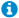# Billable Account Details

### Introduction

To see what you are being billed by LoanPro, navigate to the Billing & Statements area of the Account tab. This page is divided into six tabs, including Billable Account Details.

The Billable Account Details tab gives you a detailed daily breakdown of your company’s billable accounts. Before diving into the specifics of tab itself, it is important to understand the billable account structure. The billable account details are formatted in a table that includes columns for each billable metric. In the upper left corner of the page, there's a drop-down allowing you to select the month and year you would like to see the billable account details for.

In the top right corner, two icons let you configure how the data is presented. The hourglass toggles between a daily or cumulative view, with daily showing the details for each individual day, and cumulative adding up the totals one day at a time. Clicking the jagged line icon will switch from a table to a line graph, which can likewise show daily or cumulative sums.

#### What is a Billable Account?

“Billable Account” means the number of Accounts that are charged a monthly per unit fee. The Billable Account number will be calculated by taking the greatest number from the following metrics for the applicable Billing Cycle.

1. Live Accounts — Highest Number of Live Accounts during the Billing Cycle;
2. Customer Account Limits — If the number of Customers at the end of the Billing Cycle is more than a multiple of two and one tenth (2.1) of the sum of the number of Live Accounts plus Archived Accounts at the end of the Billing Cycle, then this value is calculated as the number of Customers at the end of the Billing Cycle divided by 2.1; otherwise this value shall equal zero (for purposes of calculating the Billable Accounts).
3. AutoPay Limits — If the number of AutoPays processed during the Billing Cycle is more than the value from subsection (a) for that Billing Cycle multiplied by four (4), then this value is calculated as the number of AutoPays processed during the Billing Cycle divided by four (4); otherwise this value shall equal zero.
4. Account Modification Tool Limits — If the number of Account modifications performed during the Billing Cycle using the Account Modification Tool is more than ten percent (10%) of the number found in subsection (a) for that Billing Cycle, then this value is calculated as the number of Account modifications performed during the Billing Cycle multiplied by ten (10); otherwise this value shall equal zero.
5. Collector Queue Limits — If the total count of occurrences Accounts are processed via a Collector Queue tool is greater than the number found in subsection (a) for that Billing Cycle multiplied by twenty-five (25), then this value is as the total count of occurrences Accounts are processed via a Collector Queue divided by twenty-five (25); otherwise this value shall equal zero.
6. Custom Form Limits — If the total number of custom forms generated during the Billing Cycle is greater than five (5) times the number in subsection (a) for that Billing Cycle, then this value is calculated as the total number of custom forms generated during the Billing Cycle divided by the number from subsection (a); otherwise this value shall equal zero.
7. Rule Evaluation Limits — If the total number of Rule Evaluations that the system performs during the Billing Cycle exceeds Seven Hundred Fifty (750) times the number found in subsection (a) above, then this shall be calculated as the total number of Rule Evaluations performed during the Billing Cycle divided by Seven Hundred Fifty (750); otherwise this value shall equal zero.

This information is available at a glance by hovering over the “” icon located to the right of the “Total Billable Accounts” header.

For any questions regarding your billable accounts, please refer to the SaaS agreement located on the My Account > Contract & Pricing > Contract tab.